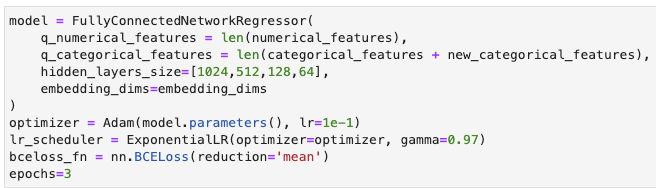Hi

I’m training a Fully Connected NN with Pytorch, and the model seems to perform very well. this is the model, and the hyper-parameters:

The Model (I’ve not changed the name to `FullyConnectedNetworkClassifier`)

As you can see, the output layer has a `sigmoid`, so my model predicts a probability

The hyper-parametersThe results

I made a terrible mistake. I didn’t realize that the both dataset’s and unbalanced. The column target has two values, `True` or `False`, and, as I said, the unbalancing comes in the form that in both dataset’s the samples with `target=False` are the 90% (10% of samples with `target=True`) of the total (both in training and validation)

So my questions:

1. My model, as it’s, … is it predicting the probability of the `True` class (`target=True`), or the False one (`target=False`)?
2. How can I parametrize `BCELoss` with `weights`? how much should be the magnitud of those `weights`? What is the in intuition of the `weight` parameter, exactly?
3. Could I use `BCEWithLogitsLoss` to work with my unbalanced dataset’s?

Best regards and thanks

Hi @jonmunm

I think there are a lot of posts regarding unbalanced datasets. For example, you can do:

1. Oversample the minority class or undersample the majority class
2. Give weights to the loss. A good starting point it 1/Frequency or 1/sqrt(frequency). I your case, I’d try to assign: `weights=[1/5 1]` so the error for False target is x5 important that the error made when the target is True.
3. Use other type of losses that focus in the top errors like the focal loss or use HEM (hard example mining). I did a fastai V2 callback sometime ago.

If you take care, you can simultaneously use all options. If you don’t know what you are doing, I’d stick to 2 and 3.

thanks for replaying @vferrer

Oversample the minority class or undersample the majority class

I’ll give a try

Give weights to the loss. A good starting point it 1/Frequency or 1/sqrt(frequency). I your case, I’d try to assign: `weights=[1/5 1]` so the error for False target is x5 important that the error made when the target is True.

This is my biggest doubt. Since my datasets are unbalanced (90% False, 10% True) …

1. The error for `False`, … shouldn’t it be 10x more important than errors for `True` like `weights=torch.tensor([0.1, 1])`

The docs about `BCELoss's weights` says “it should be a tensor of size batch_size”. In the otherside, `BCEWithLogitsLoss' pos_weight` seems to be a litle straightforward, like `pos_weight=torch.tensor()`. However, I don’t know if I’m interpreting the docs right.

A good starting point it 1/Frequency or 1/sqrt(frequency)

Could you go deeper on this, pls?

Use other type of losses that focus in the top errors like the focal loss

@jonmunm About loss weights, I didn’t read `BCEWithLogitsLoss`. I’d use `pos_weight` argument:
`criterion = torch.nn.BCEWithLogitsLoss(pos_weight=torch.tensor()` . I’d try different values between 4 and 15. Sometimes, I find more useful not to penalize the negative class too much. At the end, it’ll depend on how many false positive and false negative you want.